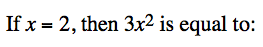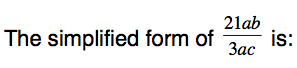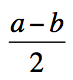Year 7 Algebraic Techniques Quiz 1
Name *
School
Country *
In the expression 3x + 2y + 4xy + 7yz the coefficient of y is:
1 point
If t = 5 and u = 7, then 2t + u is equal to:
1 point
Jack buys 3 pies at p dollars each and 2 cans of soft drink at c dollars each. If the total cost is T this can be summarised as
1 point
1 pointWhich of the following pairs does not consist of two like terms?
1 point
When 2a + 4 + 3b + 5a is fully simplified it is equivalent to
1 point
The simplified form of 4a × 3bc is:
1 point
1 pointThe value of the expression 3a + 5 when a = 2 is
1 point
Which of the following expressions does not simplify to 24b
1 point
The algebraic expression y + y + y + y is equivalent to:
1 point
5a – (2a – 7) is
1 point
If the value of 𝑎=20 and the value for 𝑏=9 then 𝑎 + 3 × 𝑏 is equal to
1 point
If a is an even number the next consecutive even number is
1 point
How many centimetres in p metres
1 point
If a = –4 and b = –6 what is the value of
1 pointIf a = –4 and b = –6 what is the value of
1 pointWhich expression is not equal to 3m?
1 point
Simplify 5a – 4 + 3a – 2
1 point
Which of the following lists contain only like terms
1 point